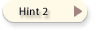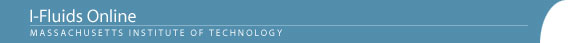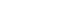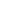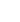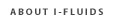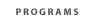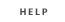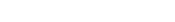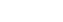2.25: Advanced Fluid Dynamics Section 1: Continuum viewpoint and the equation of motion Section 2: Static Fluids Section 3: Mass Conservation Section 4: Inviscid flow - differential approach Section 5: Control Volume Theorums Section 6: Navier-Stokes equation and viscous flow Section 7: Similarity and dimensional analysis Section 8: Boundary layers, separation and effect on drag/lift Section 9: Vorticity and circulation Section 10: Potential flows; lift, drag and thrust production Section 11: Surface tension and its effect on flows Section 12: Introduction to turbulence Back to 2.25 Home | Back to Section 5Problem 5.15: Hinged flat plate swung against wall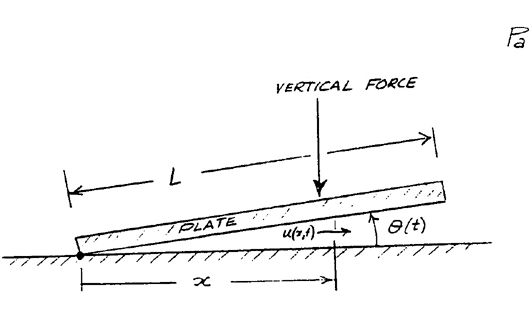A flat plate is hinged at one side to s smooth floor, as shown, and held at a small angle qo (qo<< 1) relative to the floor. The entire system is submerged in a liquid of constant density r.  At t = 0, a vertical force is applied and adjusted continually so that the rate of decrease of the plate angle q remains constant at a value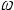: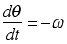. Assuming that the flow is incompressible and inviscid, derive expressions for (a) the horizontal velocity u(x,t) at point x and time t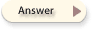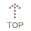(b) the x-direction force FH(t) exerted by the hinge on the floor. Assume the plate has negligible inertia.